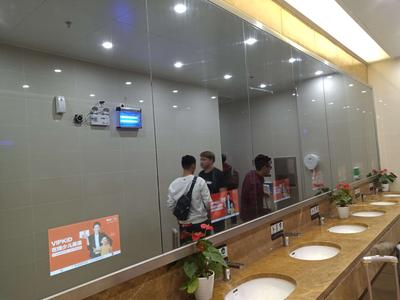# 镜显广告玻璃

 膜面/Coating surface Y=61.5 L=81.2 a= 0.6 b= -1.6 透过/Transmittance Y=35.20 L=65.70 a=-0.7 b=0.6 膜面/Coating surface Y=49.30 L=75.6 a=2.22 b= -1.1 透过/Transmittance Y=48.70 L=75.3 a= -2.00 b= -0.5